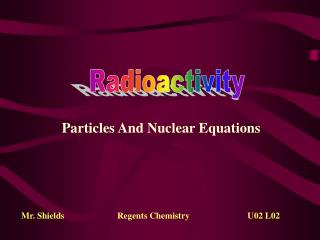Download Presentation# Radioactivity - PowerPoint PPT Presentation

Download PresentationDownload Policy: Content on the Website is provided to you AS IS for your information and personal use and may not be sold / licensed / shared on other websites without getting consent from its author. While downloading, if for some reason you are not able to download a presentation, the publisher may have deleted the file from their server.

- - - - - - - - - - - - - - - - - - - - - - - - - - - E N D - - - - - - - - - - - - - - - - - - - - - - - - - - -
##### Presentation Transcript

1. Radioactivity Particles And Nuclear Equations Mr. Shields Regents Chemistry U02 L02

2. Radioactive Emissions • There are several types of particles that can be expelled from a nucleus undergoing nuclear decay… Alpha, Beta & Gamma were First identified By Rutherford (see Table O)

3. Penetrating abilities • Particles emitted during radioactive decay have • specific penetrating power into various materials. • This provides an Indication of how much • material or “SHIELDING” would be needed to • stop the particle The greater the penetrating power the greater the health risk !

4. Particle Penetrating Power • Alpha particles are easy to stop, gamma rays are hard to stop.

5. N to P Ratio Remember the Neutron to Proton Ratio? Natural radioactivity is an attempt to correct a bad Neutron to Proton ratio It’s an attempt to get back into the “band of stability” Or to get below Atomic number 83 a nucleus may shed nuclear mass

6. Nuclear equation for alpha emission Parent Nuclide Daughter Nuclides Alpha Emission • Notice that this equation is BALANCED. Why? • Mass and Atomic number on one side of the equation • equals Mass and Atomic number on the other side

7. Alpha Emission • Why would emitting an alpha particle from U-238 • increase nuclear stability? • Atomic mass is decreased by 4 (shedding mass) • Atomic number is decreased by 2 (decreases Atomic #)

8. Beta Emission Notice that the atomic number increases by 1 But the mass stays the same Hey! How do we get an electron out of the nucleus? • Neutrons can decay to a • Proton and a beta particle. • The proton remains in the nucleus • and the beta particle is expelled.

9. Beta Emission C: n/p ratio 8/6 = 1.33 N: n/p ratio 7/7 = 1.00 • Favored by Nuclides above the “Band of Stability” • N/P ratio is too high • Beta emission Decreases the N/P ratio • neutron decays into a proton

10. Positron Emission The positron is the opposite of an beta particle (electron) Hey! So how do we get a positron out of the nucleus? • Like the Neutron, Protons can decay . • However, Proton decay into a positron • And a neutron. • The neutron remains in the nucleus • and the positron is expelled.

11. Positron Emission O: n/p ratio 7/8 = 0.88 N: n/p ratio 8/7 = 1.14 • Favored by Nuclides below the “Band of Stability” • N/P ratio is too Low • Positron emission Increases the N/P ratio • Proton decays into a neutron

12. Gamma Rays The last decay mode doesn’t actually involve a particle. Gamma radiation is actually a part of the high energy end of the EM Spectrum. Increasing Energy

13. Gamma Radiation In nuclear decay processes, gamma radiation is a way For an excited, or metastable, nucleus to get rid of excessenergy. It occurs typically as part of an alpha or beta decay process in which the nucleus is left in a high energy state.

14. Nuclear Equations • Describes the decay process. • Has a reactant side (left) and a product side (right) • starting isotope • ending isotope and emitted particle. • An “” separates the two sides of the equation • Several isotopes and their decay mode can be found in Table N.

15. Nuclear Equations • Must Demonstrate the law of conservation of mass • The SUM of the Atomic numbers and Mass numbers on each side of the arrow must be the same

16. 232Th  4He + X 90 2 Determining Unknowns in Nuclear Equations • Write the equation for the decay of Thorium-232 • Use Table N to find the decay mode: • Write the initial equation:  We need to figure out what X is

17. Alpha decay, thorium-232 228 232Th  4He + X 90 2 Conservation of Mass Number The sum of the mass numbers on the left side must equal the sum of the mass numbers on the right side.

18. Decay Equations 232Th  4He + 228X 88 90 2 Conservation of Atomic Number The sum of the atomic numbers on the left side must equal the sum of the atomic numbers on the right side.

19. 237 X 93 14C  X + 0e 14N -1 6 7  Decay of Am-241 241Am  4He + X X has to be? 2 95 So “X” is Np-237 X has to be?

20. Problems: • Write the nuclear equation for the natural decay of Co-60. (Hint: Table N)